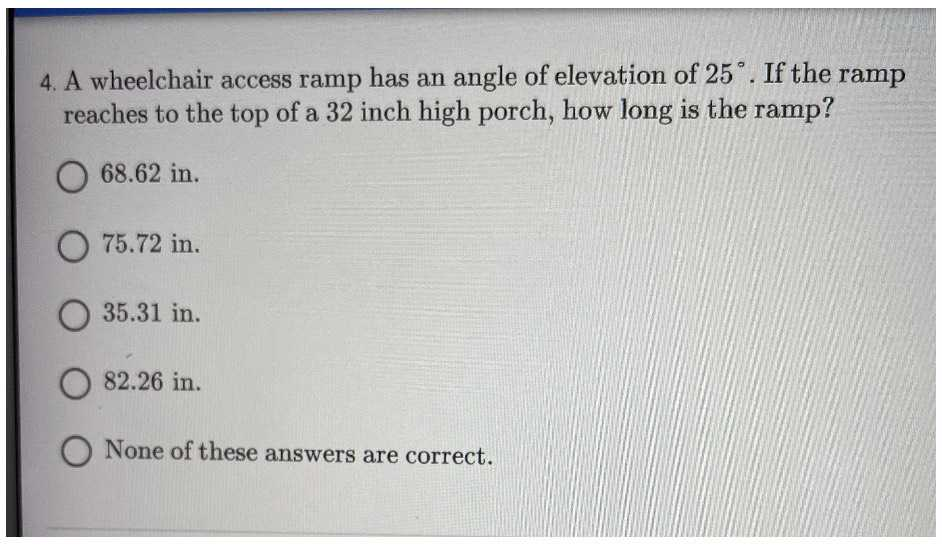### Still have math questions?

Trigonometry
Question4. A wheelchair access ramp has an angle of elevation of $$25 ^ { \circ }$$ . If the ramp reaches to the top of a $$32$$ inch high porch, how long is the ramp?

$$68.62$$ in.

$$75.72$$ in.

$$35.31$$ in.

$$82.26$$ in. None of these answers are correct.